avcontentteam — Published On November 1, 2015 and Last Modified On March 30th, 2023

## Overview

• Get an introduction to logistic regression using R and Python
• Logistic Regression is a popular classification algorithm used to predict a binary outcome
• There are various metrics to evaluate a logistic regression model such as confusion matrix, AUC-ROC curve, etc

## Introduction

Every machine learning algorithm works best under a given set of conditions. Making sure your algorithm fits the assumptions/requirements ensures superior performance. You can’t use any algorithm in any condition. For example: Have you ever tried using linear regression on a categorical dependent variable? Don’t even try! Because you won’t be appreciated for getting extremely low values of adjusted R² and F statistic.

Instead, in such situations, you should try using algorithms such as Logistic Regression, Decision Trees, SVM, Random Forest etc. To get a quick overview of these algorithms, I’ll recommend reading – Essentials of Machine Learning Algorithms.

With this post, I give you useful knowledge on Logistic Regression in R. After you’ve mastered linear regression, this comes as the natural following step in your journey. It’s also easy to learn and implement, but you must know the science behind this algorithm.

I’ve tried to explain these concepts in the simplest possible manner. Let’s get started.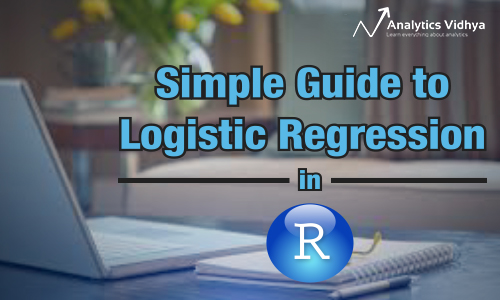## Problem Statement

HR analytics is revolutionizing the way human resources departments operate, leading to higher efficiency and better results overall. Human resources have been using analytics for years.

However, the collection, processing, and analysis of data have been largely manual, and given the nature of human resources dynamics and HR KPIs, the approach has been constraining HR. Therefore, it is surprising that HR departments woke up to the utility of machine learning so late in the game. Here is an opportunity to try predictive analytics in identifying the employees most likely to get promoted.

Practice Now

## What is Logistic Regression?

Logistic Regression is a classification algorithm. It is used to predict a binary outcome (1 / 0, Yes / No, True / False) given a set of independent variables. To represent binary/categorical outcome, we use dummy variables. You can also think of logistic regression as a special case of linear regression when the outcome variable is categorical, where we are using log of odds as dependent variable. In simple words, it predicts the probability of occurrence of an event by fitting data to a logit function.

## Derivation of Logistic Regression Equation

Logistic Regression is part of a larger class of algorithms known as Generalized Linear Model (glm). In 1972, Nelder and Wedderburn proposed this model with an effort to provide a means of using linear regression to the problems which were not directly suited for application of linear regression. Infact, they proposed a class of different models (linear regression, ANOVA, Poisson Regression etc) which included logistic regression as a special case.

The fundamental equation of generalized linear model is:

`g(E(y)) = α + βx1 + γx2`

Here, g() is the link function, E(y) is the expectation of target variable and α + βx1 + γx2 is the linear predictor ( α,β,γ to be predicted). The role of link function is to ‘link’ the expectation of y to linear predictor.

Important Points

1. GLM does not assume a linear relationship between dependent and independent variables. However, it assumes a linear relationship between link function and independent variables in logit model.
2.  The dependent variable need not to be normally distributed.
3. It does not uses OLS (Ordinary Least Square) for parameter estimation. Instead, it uses maximum likelihood estimation (MLE).
4. Errors need to be independent but not normally distributed.

Let’s understand it further using an example:

We are provided a sample of 1000 customers. We need to predict the probability whether a customer will buy (y) a particular magazine or not. As you can see, we’ve a categorical outcome variable, we’ll use logistic regression. Vidalista 20 https://tyackdentalgroup.com/vidalista

To start with logistic regression, I’ll first write the simple linear regression equation with dependent variable enclosed in a link function:

`                           g(y) = βo + β(Age)         ---- (a)`

Note: For ease of understanding, I’ve considered ‘Age’ as independent variable.

In logistic regression, we are only concerned about the probability of outcome dependent variable ( success or failure). As described above, g() is the link function. This function is established using two things: Probability of Success(p) and Probability of Failure(1-p). p should meet following criteria:

1. It must always be positive (since p >= 0)
2. It must always be less than equals to 1 (since p <= 1)

Now, we’ll simply satisfy these 2 conditions and get to the core of logistic regression. To establish link function, we’ll denote g() with ‘p’ initially and eventually end up deriving this function.

Since probability must always be positive, we’ll put the linear equation in exponential form. For any value of slope and dependent variable, exponent of this equation will never be negative.

`p = exp(βo + β(Age)) = e^(βo + β(Age))    ------- (b)`

To make the probability less than 1, we must divide p by a number greater than p. This can simply be done by:

`p  =  exp(βo + β(Age)) / exp(βo + β(Age)) + 1   =   e^(βo + β(Age)) / e^(βo + β(Age)) + 1    ----- (c)`

Using (a), (b) and (c), we can redefine the probability as:

`              p = e^y/ 1 + e^y           --- (d)`

where p is the probability of success. This (d) is the Logit Function

If p is the probability of success, 1-p will be the probability of failure which can be written as:

`q = 1 - p = 1 - (e^y/ 1 + e^y)    --- (e)`

where q is the probability of failure

On dividing, (d) / (e), we get,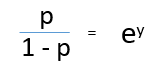After taking log on both side, we get,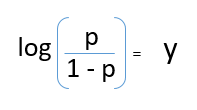log(p/1-p) is the link function. Logarithmic transformation on the outcome variable allows us to model a non-linear association in a linear way.

After substituting value of y, we’ll get: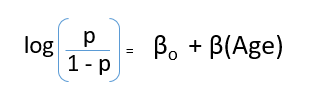This is the equation used in Logistic Regression. Here (p/1-p) is the odd ratio. Whenever the log of odd ratio is found to be positive, the probability of success is always more than 50%. A typical logistic model plot is shown below. You can see probability never goes below 0 and above 1.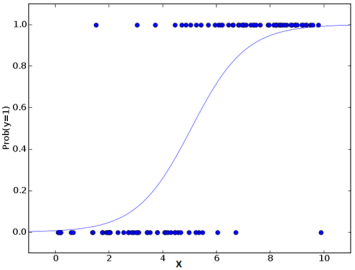## Performance of Logistic Regression Model

To evaluate the performance of a logistic regression model, we must consider few metrics. Irrespective of tool (SAS, R, Python) you would work on, always look for:

1. AIC (Akaike Information Criteria) – The analogous metric of adjusted R² in logistic regression is AIC. AIC is the measure of fit which penalizes model for the number of model coefficients. Therefore, we always prefer model with minimum AIC value.

2. Null Deviance and Residual Deviance – Null Deviance indicates the response predicted by a model with nothing but an intercept. Lower the value, better the model. Residual deviance indicates the response predicted by a model on adding independent variables. Lower the value, better the model.

3. Confusion Matrix: It is nothing but a tabular representation of Actual vs Predicted values. This helps us to find the accuracy of the model and avoid overfitting. This is how it looks like:Source: (plug – n – score)

You can calculate the accuracy of your model with: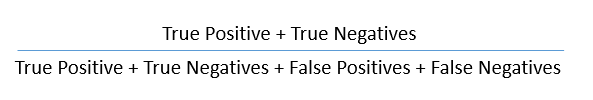From confusion matrix, Specificity and Sensitivity can be derived as illustrated below: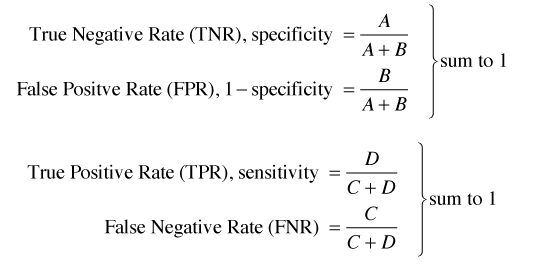Specificity and Sensitivity plays a crucial role in deriving ROC curve.

4. ROC Curve: Receiver Operating Characteristic(ROC) summarizes the model’s performance by evaluating the trade offs between true positive rate (sensitivity) and false positive rate(1- specificity). For plotting ROC, it is advisable to assume p > 0.5 since we are more concerned about success rate. ROC summarizes the predictive power for all possible values of p > 0.5.  The area under curve (AUC), referred to as index of accuracy(A) or concordance index, is a perfect performance metric for ROC curve. Higher the area under curve, better the prediction power of the model. Below is a sample ROC curve. The ROC of a perfect predictive model has TP equals 1 and FP equals 0. This curve will touch the top left corner of the graph.Note: For model performance, you can also consider likelihood function. It is called so, because it selects the coefficient values which maximizes the likelihood of explaining the observed data. It indicates goodness of fit as its value approaches one, and a poor fit of the data as its value approaches zero.

## Logistic Regression Model in R and Python

The R code is provided below but if you’re a Python user, here’s an awesome code window to build your logistic regression model. No need to open Jupyter – you can do it all here:

Considering the availability, I’ve built this model on our practice problem – Dressify data set. You can download it here.

Without going deep into feature engineering, here’s the script of simple logistic regression model:

`setwd('C:/Users/manish/Desktop/dressdata')`
```#load data

```
```#create training and validation data from given data
install.packages('caTools')
library(caTools)

```
```set.seed(88)
split <- sample.split(train\$Recommended, SplitRatio = 0.75)

```
```#get training and test data
dresstrain <- subset(train, split == TRUE)
dresstest <- subset(train, split == FALSE)

```
```#logistic regression model
model <- glm (Recommended ~ .-ID, data = dresstrain, family = binomial)
summary(model)```
`predict <- predict(model, type = 'response')`
```#confusion matrix
table(dresstrain\$Recommended, predict > 0.5)```
```#ROCR Curve
library(ROCR)
ROCRpred <- prediction(predict, dresstrain\$Recommended)
ROCRperf <- performance(ROCRpred, 'tpr','fpr')
plot(ROCRperf, colorize = TRUE, text.adj = c(-0.2,1.7))```
```#plot glm
library(ggplot2)
ggplot(dresstrain, aes(x=Rating, y=Recommended)) + geom_point() +
stat_smooth(method="glm", family="binomial", se=FALSE)```

This data require lots of cleaning and feature engineering. The scope of this article restricted me to keep the example focused on building logistic regression model. This data is available for practice. I’d recommend you to work on this problem. There’s a lot to learn.

## End Notes

By now, you would know the science behind logistic regression. I’ve seen many times that people know the use of this algorithm without actually having knowledge about its core concepts. I’ve tried my best to explain this part in simplest possible manner. The example above only shows the skeleton of using logistic regression in R. Before actually approaching to this stage, you must invest your crucial time in feature engineering.

Furthermore, I’d recommend you to work on this problem set. You’d explore things which you might haven’t faced before.

### If you like what you just read & want to continue your analytics learning, subscribe to our emails, follow us on twitter or like our facebook page.## 21 thoughts on "Simple Guide to Logistic Regression in R and Python"###### Thanh Le says:November 02, 2015 at 5:12 am
Very nice article but the figure of confusion matrix does not match with the specificity/sensitivity formulas. Should change to TNR = D/C+D ; TPR = A/A+B Reply###### Analytics Vidhya Content Team says:November 02, 2015 at 5:34 am
Hello Thanh Le I too just noticed that. Have made the change. Thank you Reply###### prakash says:November 02, 2015 at 6:41 am
Hi Manish, Awesome Article; In this case in the training dataset the deviance are as follows: Null deviance: 366.42 on 269 degrees of freedom Residual deviance: 143.20 on 140 degrees of freedom AIC: 403.2 And the minimum AIC is the better the model is going to be that we know; Can you suggest some way to say whether this AIC is good enough and how do we justify that there will not be any good possible model having lower AIC; And also I want to know some more details about this criterion to check the model; Number of Fisher Scoring iterations: 18 Reply###### Analytics Vidhya Content Team says:November 02, 2015 at 10:20 am
Hi Prakash Thanks for your appreciation. Kudos to my team indeed. You should not consider AIC criterion in isolation. Like, you've run this model and got some AIC value. You must be thinking, what to do next? You can't do anything unless you build another model and then compare their AIC values. Model with lower AIC should be your choice. Always. Number of Fisher Scoring iterations is a derivative of Newton-Raphson algorithm which proposes how the model was estimated. In your case, it can be interpreted as, Fisher scoring algorithm took 18 iterations to perform the fit. This metric doesn't tell you anything which you must know. It just confirms the model convergence. That's it. Reply###### rishi says:November 02, 2015 at 9:59 pm
Nice ...But could you also add up how to decide the cut off for logistic regression in R.. I mean the intersection of sensitivity and specifity plot. Reply###### prakash says:November 03, 2015 at 12:22 pm
Manish, I am working on a project where I am building a model on transaction-wise data; there are some 5000 customer and among them 1200 churned till data; and total transaction is 4.5 Lacs out of that 1 lacs is for the churned and rest is for non churned; Now i am trying to build the model marking those 1 Lacs as 1 and rest all as 0; and took some sample of that; say of 120000 rows; here 35 K rows have marked as 1 and rest all 0; the ratio > 15% so we can go for logistic; (as i know) now when i built the model transaction wise this accuracy from confusion matrix is coming as 76% and when we applt the model in the entire dataset, and aggregated customerwise by doing customerwise averaging the predicted transaction probabilities; and in this case out of 5000 customer, A1P1=950, A1P0=250, A0P0= 3600, A0P1=200 and hence accuracy is 91%; do u think i can feel that this model is pretty good?? in this case i made 5-6 models and the minimum AIC and corresponding tests gave me the confidence to select this model; Please share ur views and hope I am able to convey you my words; https://in.linkedin.com/in/prakashmathsiitg Reply###### Jagz says:November 04, 2015 at 9:41 am###### Analytics Vidhya Content Team says:November 04, 2015 at 10:56 am###### Pruthviraj S PhanendraKumar says:November 10, 2015 at 4:45 am
Hi Manish Very good article to understand the fundamental behind the logistic regressing. Nice explanation of the mathematics behind the scenes. Great work! Reply###### Sadashiv says:November 16, 2015 at 8:00 am
Hi Manish, Thanks for great article. Would like to understand how should I read the output of summary function. How does it helps in selecting significant variables ? Thanks for your time. Reply###### Rajanna says:November 18, 2015 at 6:48 am
Hi , Its a nice notes on logistic regression, Thanks for sharing. Can you please also include how to use MACRO economic factors in this model. For example I have 10 k customers demographic data; credit number age salary income # ofchildren 2323323232 32 23k 3l 2 545433433 27 45k 6l 3 ...... so on I am not sure how to use macro economic factors like un-employment rate , GDP,.... in this logistic model. because the macro eco data is time dependent. PLease help me to work on this type of data. Thanks in advance Rajanna . Reply###### Wenjing says:December 27, 2015 at 9:11 am
Hi Manish, Thanks for the case study! That is a great learning experience! One question on a series of dummy variable that is created in the dataset. What is the purpose for that? As those variables created are not used in the random forest modeling process in the next step. Many thanks! Reply###### Shubham Rohatgi says:April 09, 2016 at 5:41 am
Hi Manish, Great article indeed :) Kudos to your team. You can also add Wald statistics → used to test the significance of the individual coefficients and pseudo R sqaures like R^2 logit = {-2LL(of null model) - (-2LL(of proposed model)}/ (-2LL (of null model)) → used to check the overall significance of the model. #Note → here LL means log likelihood value. Reply###### Vishal says:May 03, 2016 at 1:14 pm
Hello, I see that the thread for practice problem 1 is closed and the dataset is not not available. Please provide the dataset for practice. Thank you Reply###### Sanket Agrawal says:May 21, 2016 at 6:16 am
Hi Manish, I didn't get the proper concept of set.seed() in implementing logistic regression. Can you please tell me why it is used in the code? Regards, Sanket Reply###### Aritra chatterjee says:August 09, 2016 at 1:17 pm
Inwas studying ols in edx and i was looking better explanation in terms of selection of threshold value. Thank you Manish, you made my day. Thanks Aritra chatterjee Reply###### Abhishek Jain says:August 11, 2016 at 9:11 am
I ran 10 fold Cross validation on titanic survivor data using logit model. I got varying values of accuracy (computed using confusion matrix) and their respective AIC: Accuracy AIC 1 0.797 587.4 2 0.772 577.3 3 0.746 587.7 4 0.833 596.1 5 0.795 587.7 6 0.844 600.3 7 0.811 578.8 8 0.703 568.4 9 0.768 584.6 10 0.905 614.8 How do we decide if this is a good model? Which criteria should be given weight while deciding that - Accuracy or AIC? Reply###### Nitin says:September 06, 2016 at 11:27 am